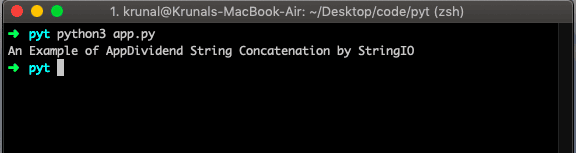# Python String Concatenation: How to Join Strings in Python

0
7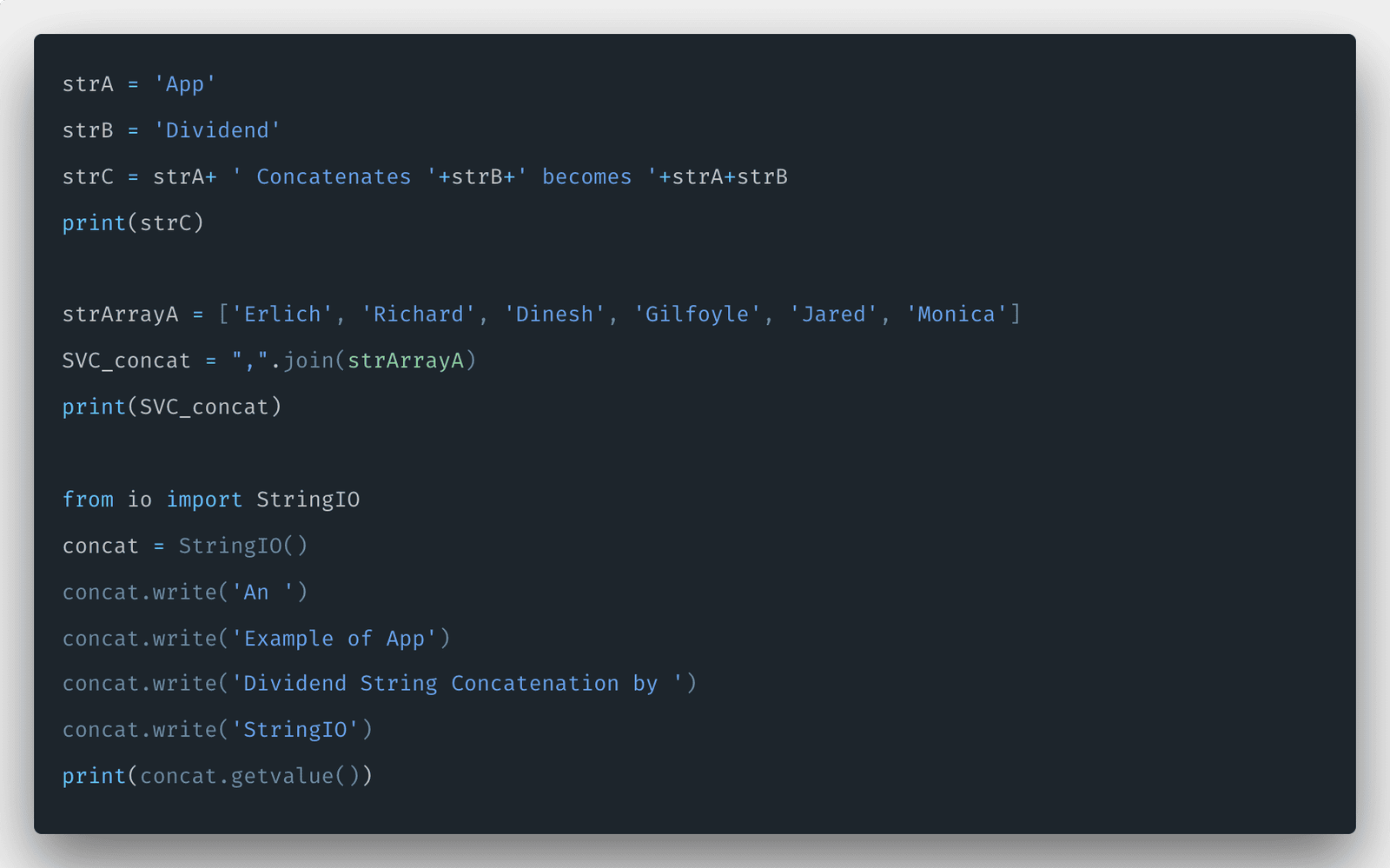Python string concat() is an inbuilt function that concatenates two or more strings. The combining strings process is referred to as concatenation. Strings in Python are immutable. There are a few ways of doing string concatenation in python depending on what you’re trying to achieve in your project.

The simplest and most common approach is to use the plus symbol (+) to add multiple strings together. Merely place a (+) between as many strings as you want to join together.

## Python string concatenation

One common task you will need to accomplish with any language involves merging or combining two or more strings.

The best way to describe it is when you take two separate strings stored by the interpreter and merge them so that they become one.

For instance, one string would be “App” and the other would be “Dividend.” When you use concatenation to combine strings, it becomes one string, and in our case, it is “AppDividend.”

## An example of + operator to concatenate strings

In the first example, let us see the plus(+) operator concatenate the two strings.

```# app.py

strA = 'App'
strB = 'Dividend'
strC = strA+ ' Concatenates '+strB+' becomes '+strA+strB

print(strC)```

In the third line in this code, the concatenation between the strings is performed, and when the interpreter executes it, it creates the new string. Run the file and see the output.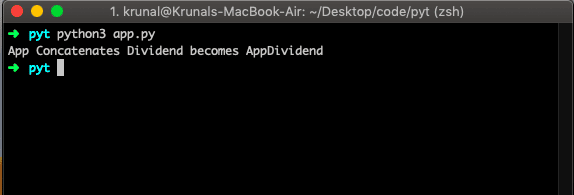One note here is that Python does not do implicit string conversion. If you try to concatenate a string with a non-string type, Python will raise a TypeError exception.

If you do not know what exception is then check out my this article on Exception Handling in Python.

## Concatenating Strings by a .join() method in Python

There is another powerful method to concate the string which is called join() method.

It is an efficient way of concatenating strings in Python if you have many strings.

One thing here to note that the join() method works with iterators like list, tuple, etc.

Let us take an example of List and see how the join() method works in Python.

```# app.py

strArrayA = ['Erlich', 'Richard', 'Dinesh', 'Gilfoyle', 'Jared', 'Monica']
SVC_concat = " ".join(strArrayA)

print(SVC_concat)```

My recommendation is to place many strings into the list and then use the join() method to combine them by using a separator. Here, we join each element of the strings list with space.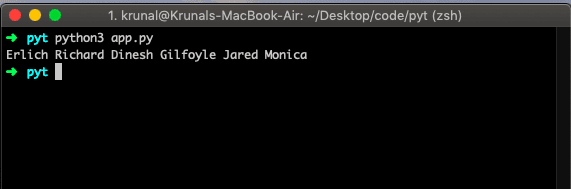Now let us take an example of joining each element of the strings list with a comma (,) and call a .join() method on it rather than the strings list.

```# app.py

strArrayA = ['Erlich', 'Richard', 'Dinesh', 'Gilfoyle', 'Jared', 'Monica']
SVC_concat = ",".join(strArrayA)```

#### Output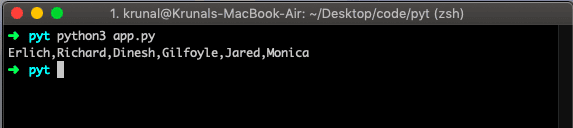## StringIO for concatenating strings

First, we have imported the StringIO module. We can use the StringIO module to concat the strings. Let us see the following example.

```# app.py

from io import StringIO
concat = StringIO()

concat.write('An ')
concat.write('Example of App')
concat.write('Dividend String Concatenation by ')
concat.write('StringIO')

print(concat.getvalue())```

#### Output